# R S Aggarwal Solutions for Class 10 Maths Chapter 5 Arithmetic Progression Exercise 5B

R S Aggarwal Solutions for Class 10 Maths exercise 5b are given here. Exercise 5b questions are solved by subject experts at BYJU’S, explaining the method of solving arithmetic mean between two numbers. To score well in exams, students are advised to solve all the questions of this exercise. Download pdf of Class 10 Maths Chapter 5 R S Aggarwal Solutions and start practicing.

## Download PDF of R S Aggarwal Solutions for Class 10 Maths Chapter 5 Arithmetic Progression Exercise 5B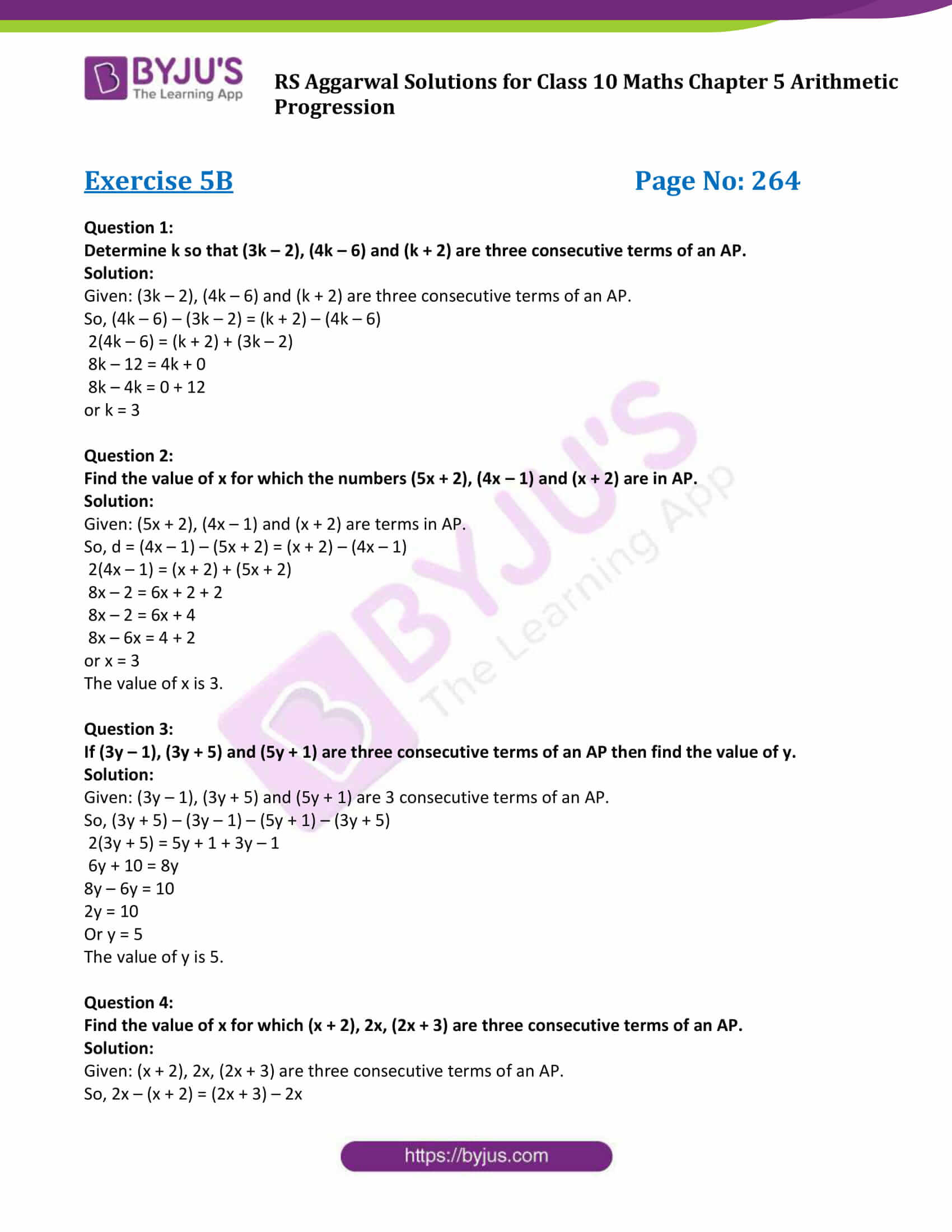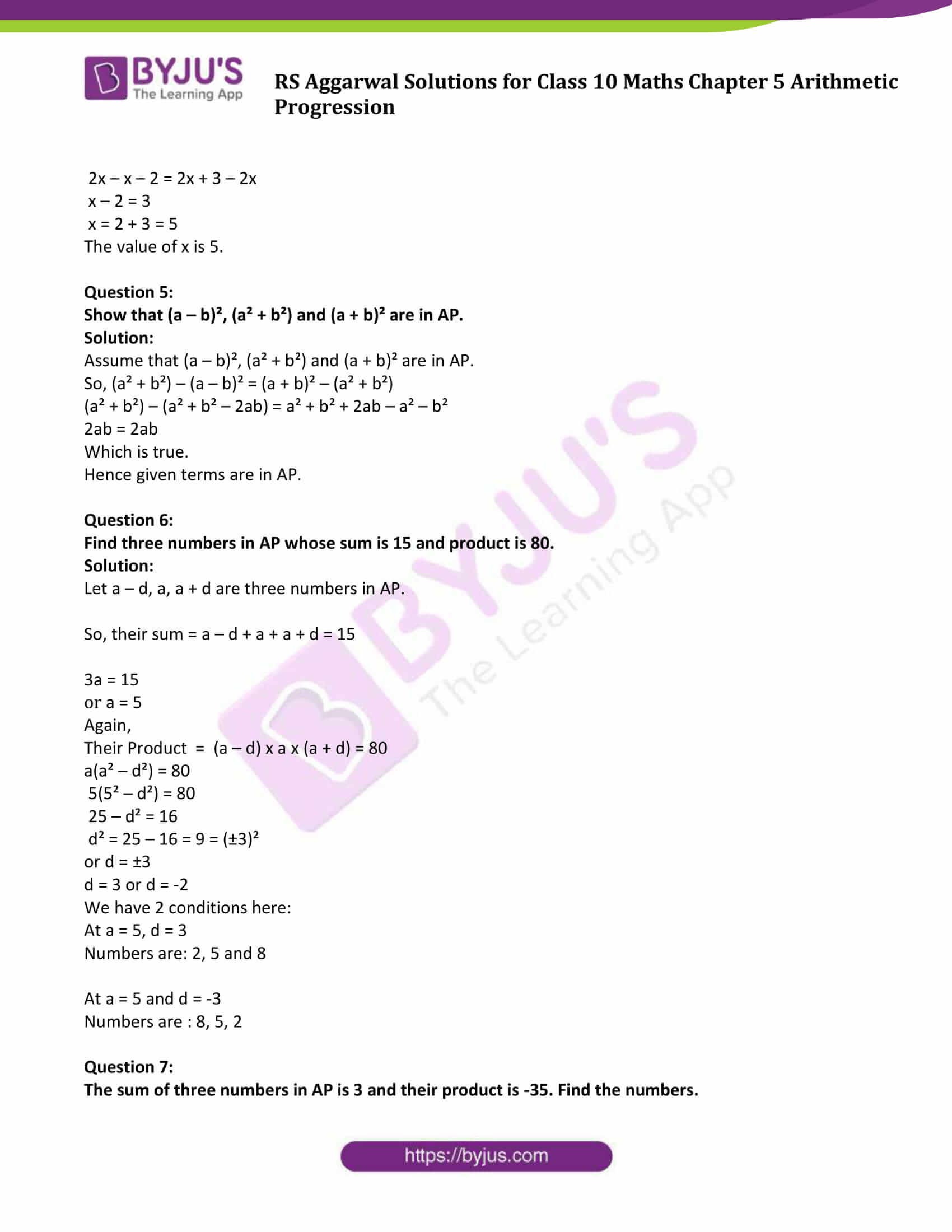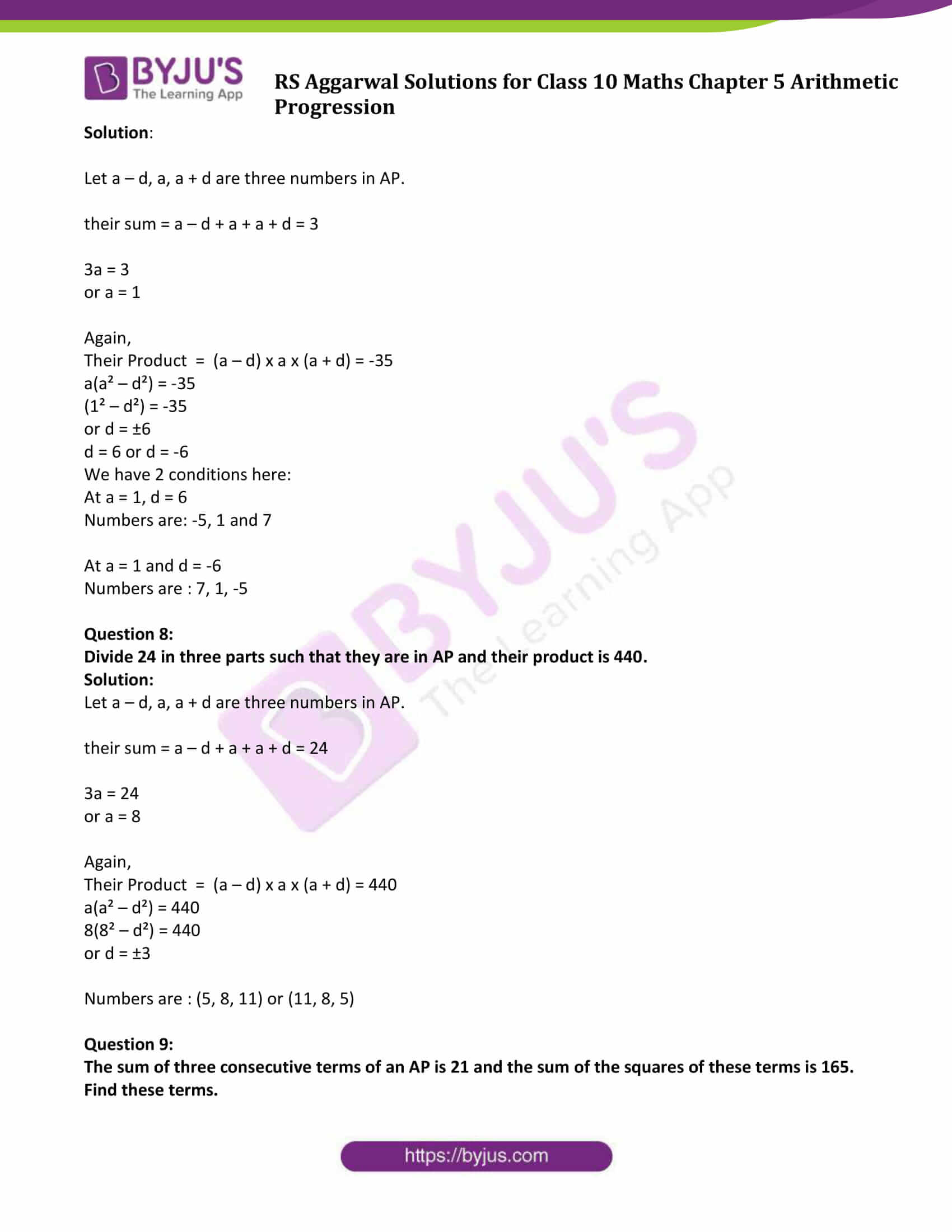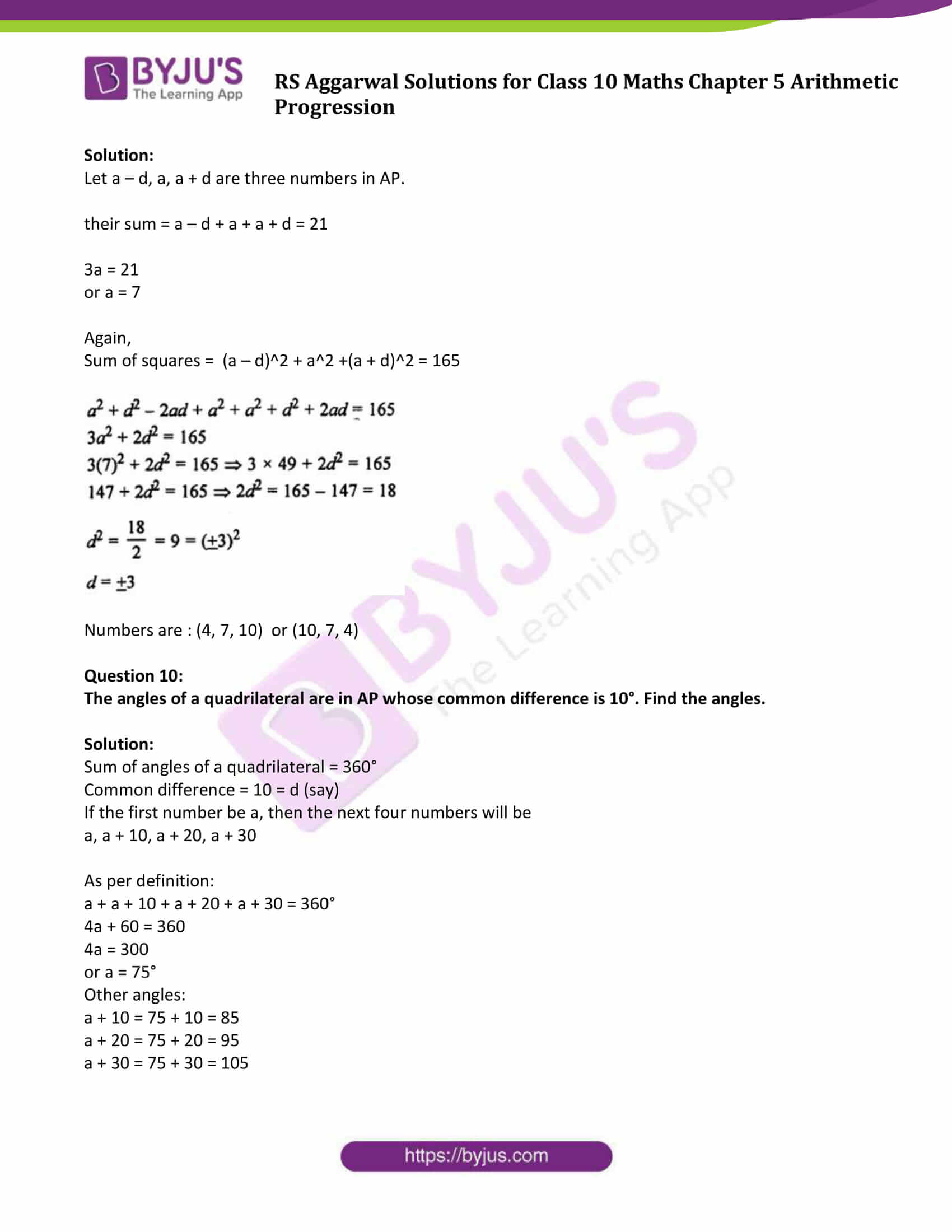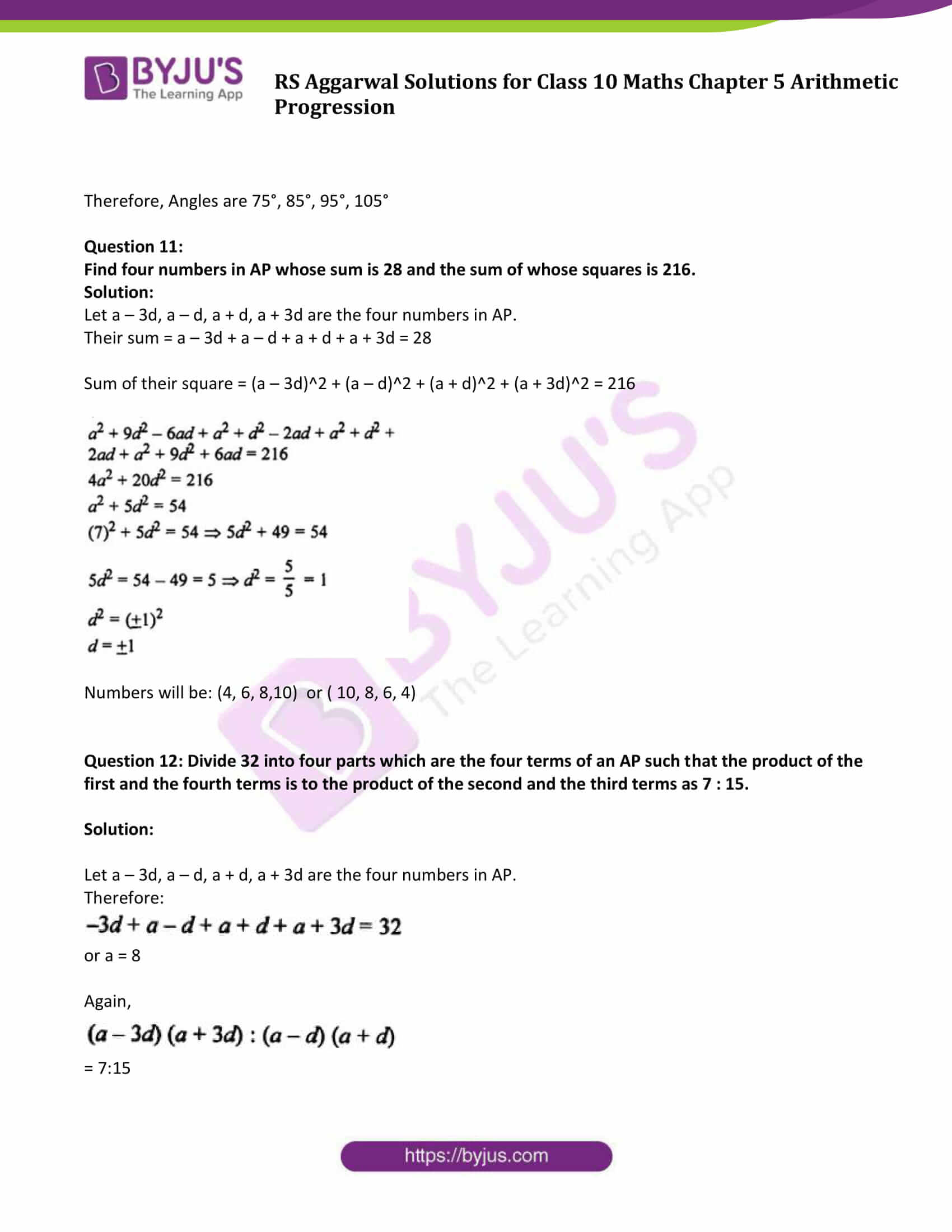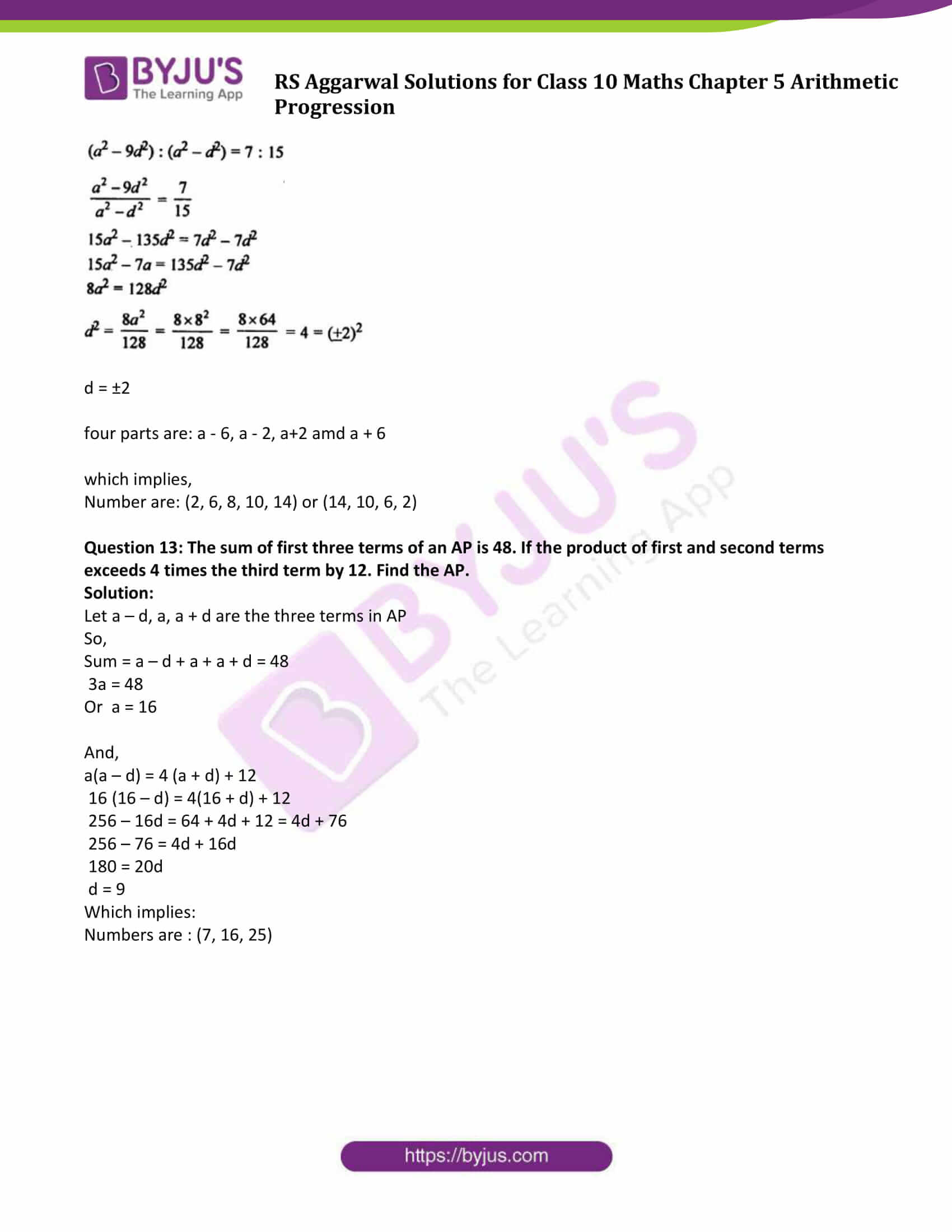### Access other exercise solutions of Class 10 Maths Chapter 5 Arithmetic Progression

Exercise 5A Solutions: 30 Questions (Short Answers)

Exercise 5C Solutions: 15 Questions (Short Answers)

Exercise 5D Solutions: 20 Questions (Long Answers)

## Exercise 5B Page No: 264

Question 1:

Determine k so that (3k – 2), (4k – 6) and (k + 2) are three consecutive terms of an AP.

Solution:

Given: (3k – 2), (4k – 6) and (k + 2) are three consecutive terms of an AP.

So, (4k – 6) – (3k – 2) = (k + 2) – (4k – 6)

2(4k – 6) = (k + 2) + (3k – 2)

8k – 12 = 4k + 0

8k – 4k = 0 + 12

or k = 3

Question 2:

Find the value of x for which the numbers (5x + 2), (4x – 1) and (x + 2) are in AP.

Solution:

Given: (5x + 2), (4x – 1) and (x + 2) are terms in AP.

So, d = (4x – 1) – (5x + 2) = (x + 2) – (4x – 1)

2(4x – 1) = (x + 2) + (5x + 2)

8x – 2 = 6x + 2 + 2

8x – 2 = 6x + 4

8x – 6x = 4 + 2

or x = 3

The value of x is 3.

Question 3:

If (3y – 1), (3y + 5) and (5y + 1) are three consecutive terms of an AP then find the value of y.

Solution:

Given: (3y – 1), (3y + 5) and (5y + 1) are 3 consecutive terms of an AP.

So, (3y + 5) – (3y – 1) – (5y + 1) – (3y + 5)

2(3y + 5) = 5y + 1 + 3y – 1

6y + 10 = 8y

8y – 6y = 10

2y = 10

Or y = 5

The value of y is 5.

Question 4:

Find the value of x for which (x + 2), 2x, (2x + 3) are three consecutive terms of an AP.

Solution:

Given: (x + 2), 2x, (2x + 3) are three consecutive terms of an AP.

So, 2x – (x + 2) = (2x + 3) – 2x

2x – x – 2 = 2x + 3 – 2x

x – 2 = 3

x = 2 + 3 = 5

The value of x is 5.

Question 5:

Show that (a – b)², (a² + b²) and (a + b)² are in AP.

Solution:

Assume that (a – b)², (a² + b²) and (a + b)² are in AP.

So, (a² + b²) – (a – b)² = (a + b)² – (a² + b²)

(a² + b²) – (a² + b² – 2ab) = a² + b² + 2ab – a² – b²

2ab = 2ab

Which is true.

Hence given terms are in AP.

Question 6:

Find three numbers in AP whose sum is 15 and product is 80.

Solution:

Let a – d, a, a + d are three numbers in AP.

So, their sum = a – d + a + a + d = 15

3a = 15

or a = 5

Again,

Their Product = (a – d) x a x (a + d) = 80

a(a² – d²) = 80

5(5² – d²) = 80

25 – d² = 16

d² = 25 – 16 = 9 = (±3)²

or d = ±3

d = 3 or d = -2

We have 2 conditions here:

At a = 5, d = 3

Numbers are: 2, 5 and 8

At a = 5 and d = -3

Numbers are : 8, 5, 2

Question 7:

The sum of three numbers in AP is 3 and their product is -35. Find the numbers.

Solution:

Let a – d, a, a + d are three numbers in AP.

their sum = a – d + a + a + d = 3

3a = 3

or a = 1

Again,

Their Product = (a – d) x a x (a + d) = -35

a(a² – d²) = -35

(1² – d²) = -35

or d = ±6

d = 6 or d = -6

We have 2 conditions here:

At a = 1, d = 6

Numbers are: -5, 1 and 7

At a = 1 and d = -6

Numbers are : 7, 1, -5

Question 8:

Divide 24 in three parts such that they are in AP and their product is 440.

Solution:

Let a – d, a, a + d are three numbers in AP.

their sum = a – d + a + a + d = 24

3a = 24

or a = 8

Again,

Their Product = (a – d) x a x (a + d) = 440

a(a² – d²) = 440

8(8² – d²) = 440

or d = ±3

Numbers are : (5, 8, 11) or (11, 8, 5)

Question 9:

The sum of three consecutive terms of an AP is 21 and the sum of the squares of these terms is 165. Find these terms.

Solution:

Let a – d, a, a + d are three numbers in AP.

their sum = a – d + a + a + d = 21

3a = 21

or a = 7

Again,

Sum of squares = (a – d)^2 + a^2 +(a + d)^2 = 165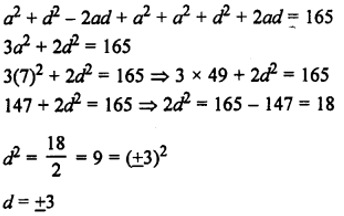Numbers are : (4, 7, 10) or (10, 7, 4)

Question 10:

The angles of a quadrilateral are in AP whose common difference is 10°. Find the angles.

Solution:

Sum of angles of a quadrilateral = 360°

Common difference = 10 = d (say)

If the first number be a, then the next four numbers will be

a, a + 10, a + 20, a + 30

As per definition:

a + a + 10 + a + 20 + a + 30 = 360°

4a + 60 = 360

4a = 300

or a = 75°

Other angles:

a + 10 = 75 + 10 = 85

a + 20 = 75 + 20 = 95

a + 30 = 75 + 30 = 105

Therefore, Angles are 75°, 85°, 95°, 105°

Question 11:

Find four numbers in AP whose sum is 28 and the sum of whose squares is 216.

Solution:

Let a – 3d, a – d, a + d, a + 3d are the four numbers in AP.

Their sum = a – 3d + a – d + a + d + a + 3d = 28

Sum of their square = (a – 3d)^2 + (a – d)^2 + (a + d)^2 + (a + 3d)^2 = 216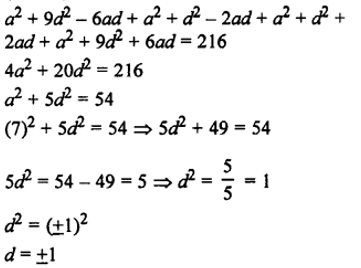Numbers will be: (4, 6, 8,10) or ( 10, 8, 6, 4)

Question 12: Divide 32 into four parts which are the four terms of an AP such that the product of the first and the fourth terms is to the product of the second and the third terms as 7 : 15.

Solution:

Let a – 3d, a – d, a + d, a + 3d are the four numbers in AP.

Therefore: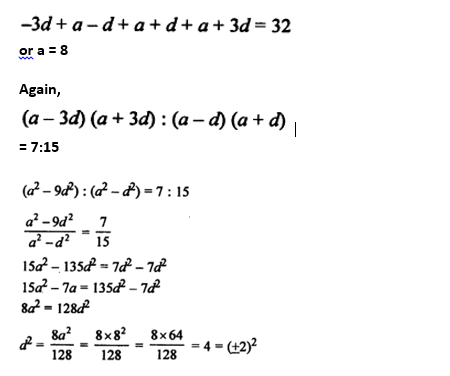d = ±2

four parts are: a – 6, a – 2, a+2 amd a + 6

which implies,

Number are: (2, 6, 8, 10, 14) or (14, 10, 6, 2)

Question 13: The sum of first three terms of an AP is 48. If the product of first and second terms exceeds 4 times the third term by 12. Find the AP.

Solution:

Let a – d, a, a + d are the three terms in AP

So,

Sum = a – d + a + a + d = 48

3a = 48

Or a = 16

And,

a(a – d) = 4 (a + d) + 12

16 (16 – d) = 4(16 + d) + 12

256 – 16d = 64 + 4d + 12 = 4d + 76

256 – 76 = 4d + 16d

180 = 20d

d = 9

Which implies:

Numbers are : (7, 16, 25)

## R S Aggarwal Solutions for Class 10 Maths Chapter 5 Arithmetic Progression Exercise 5B

Class 10 Maths Chapter 5 Arithmetic Progression Exercise 5B is based on following topics:

• Arithmetic Mean
• Arithmetic mean between two numbers

R S Aggarwal Solutions for Class 10 Maths, students will be able to clear all their doubts related to arithmetic progression to score good marks in the exams.